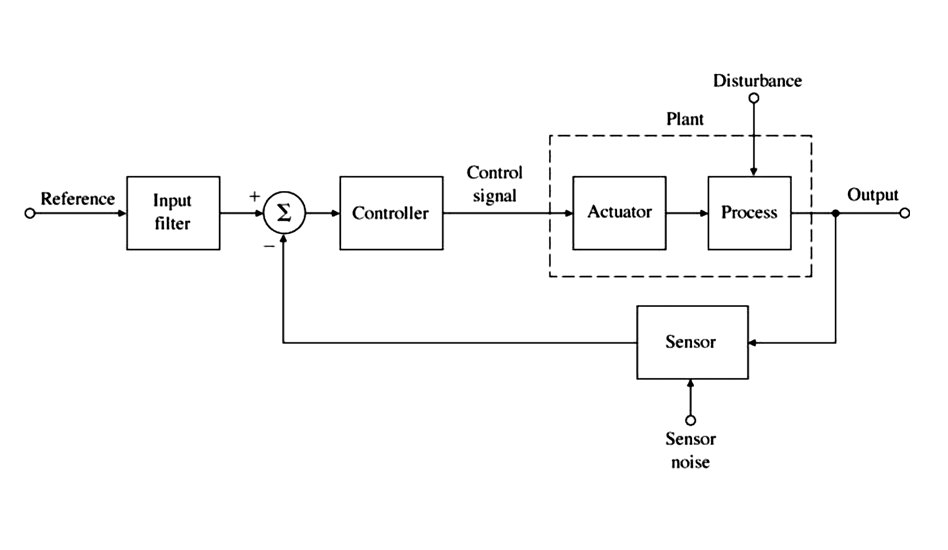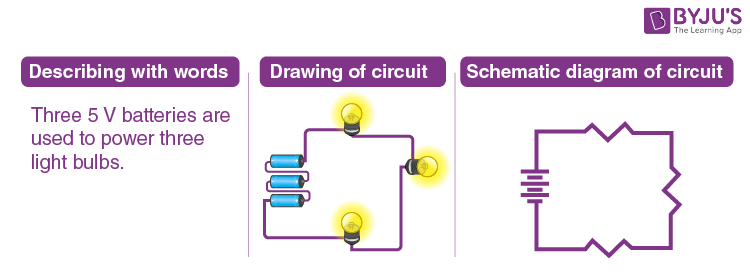# Schematic Diagram Explained: A Comprehensive Guide to Wiring Diagrams

When tackling a wiring job, the first step is to understand the connections that need to be made. To do that, you need to have an understanding of schematic diagrams and how they work. Schematic diagrams are like road maps for electronics and wiring, showing components and their connections in a visual manner. They are typically used as part of the design process to help plan and document an electrical system or circuit. In this guide, we take a look at what schematic diagrams are and how they’re used.

## What is a Schematic Diagram?

A schematic diagram (also known as a circuit diagram or wiring diagram) is a visual representation of an electrical circuit. It shows the components of the circuit as well as their connections. The schematic diagram uses symbols to represent the components in the circuit, and lines to represent the wires connecting them. Schematic diagrams are essential in helping designers and engineers plan and debug circuits.

## Components of a Schematic Diagram

Understanding the components of a schematic diagram is essential for anyone who needs to use them to design or troubleshoot an electronic circuit. Here are the main components of a schematic diagram:

• Symbols: Symbols are used to represent the different components of the circuit. These symbols vary from one diagram to the next, but the most common types include resistors, capacitors, transistors, relays, switches, and diodes.

• Connectors: Connectors are used to represent the physical connection between two components in the circuit.

• Wires: Wires are represented by lines on the diagram and indicate the path in which current will flow through the circuit.

• Ground: The ground symbol is used to represent a common connection point for all components in the circuit.

## How to Read a Schematic Diagram?

Reading a schematic diagram is fairly simple once you understand the symbols and their meanings. You should start by familiarizing yourself with the symbols and labels used in the diagram. Once you know what the symbols mean, you can then begin to interpret the diagram.

The basic structure of a schematic diagram is that it shows the components of the circuit in a logical order. This makes it easier to understand how the circuit works. To read the diagram, start by tracing the current as it flows through the circuit. Look for the power source (battery) and follow the current as it flows from one component to the next. As you do this, take note of the connections between each component.

## Benefits of Using Schematic Diagrams

Schematic diagrams are incredibly useful when it comes to visualizing and understanding an electronic circuit. Here are some of the main benefits of using them:

• Easy to Understand: Schematic diagrams are much easier to understand than written descriptions of a circuit. The symbols used in the diagram make it easy to visualize the components and connections in the circuit.

• Design & Troubleshooting: Schematic diagrams are invaluable when it comes to designing and troubleshooting circuits. They provide a visual map of the circuit which makes it much easier to spot problems or areas that need improvement.

• Simplicity: Schematic diagrams are much simpler to draw than an actual circuit board. This makes them ideal for quickly sketching out and documenting a circuit.

## Conclusion

Schematic diagrams are a valuable tool for anyone who needs to design and troubleshoot electronic circuits. They are easy to understand and can help simplify the design and debugging process. By understanding what schematic diagrams are and how to use them, you’ll have a much easier time tackling any wiring job.How To Read Car Wiring Diagrams Short Beginners Version Rustyautos ComSchematic Diagram MapAppendix Electronics Construction Primer Mcgraw Hill Education Access EngineeringElectrical Drawings And Schematics OverviewDraw An Appropriate Schematic Diagram Showing Common Domestic Circuits And Discuss The Importance Of Fuse Why Is It That A Burnt Out Should Be Replaced By Another Identical RatingGenerating Service Component Definition Language From Metadata Diagram Schematic And Image 03What Is The Meaning Of Schematic Diagram Sierra CircuitsCircuit Diagram And Its Components Explanation With SymbolsWiring Diagram A Comprehensive Guide Edrawmax OnlineSchematic Diagram Of The Sequence Events In Experiment 1 This ScientificDifference Between Pictorial And Schematic Diagrams Lucidchart BlogWiring Diagram Everything You Need To Know AboutWhat Is A Schematic DiagramSchematic Of Experimental Procedure Diagram Depicting The ScientificDifference Between Pictorial And Schematic Diagrams Lucidchart BlogWiring Diagram Templates